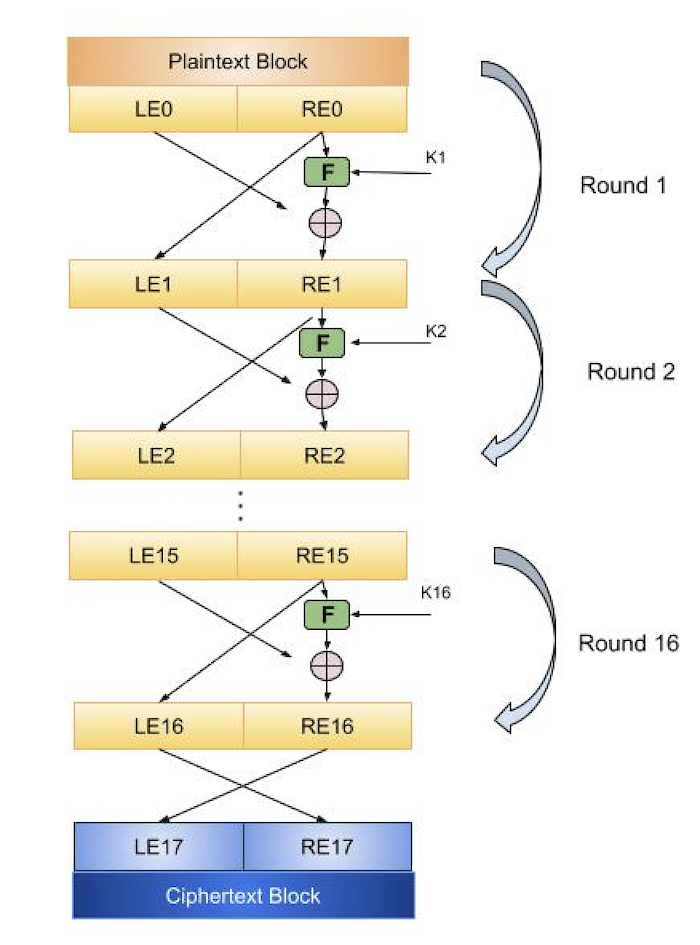# Feistel Block Cipher

Feistel Cipher is not a specific scheme of block cipher. It is a design model from which many different block ciphers are derived. DES is just one example of a Feistel Cipher. A cryptographic system based on Feistel cipher structure uses the same algorithm for both encryption and decryption.

## Encryption Process

The encryption process uses the Feistel structure consisting multiple rounds of processing of the plaintext, each round consisting of a “substitution” step followed by a permutation step.

Feistel Structure is shown in the following illustration −• The input block to each round is divided into two halves that can be denoted as L and R for the left half and the right half.

• In each round, the right half of the block, R, goes through unchanged. But the left half, L, goes through an operation that depends on R and the encryption key. First, we apply an encrypting function ‘f’ that takes two input − the key K and R. The function produces the output f(R,K). Then, we XOR the output of the mathematical function with L.

• In real implementation of the Feistel Cipher, such as DES, instead of using the whole encryption key during each round, a round-dependent key (a subkey) is derived from the encryption key. This means that each round uses a different key, although all these subkeys are related to the original key.

• The permutation step at the end of each round swaps the modified L and unmodified R. Therefore, the L for the next round would be R of the current round. And R for the next round be the output L of the current round.

• Above substitution and permutation steps form a ‘round’. The number of rounds are specified by the algorithm design.

• Once the last round is completed then the two sub blocks, ‘R’ and ‘L’ are concatenated in this order to form the ciphertext block.

The difficult part of designing a Feistel Cipher is selection of round function ‘f’. In order to be unbreakable scheme, this function needs to have several important properties that are beyond the scope of our discussion.

## Decryption Process

The process of decryption in Feistel cipher is almost similar. Instead of starting with a block of plaintext, the ciphertext block is fed into the start of the Feistel structure and then the process thereafter is exactly the same as described in the given illustration.

The process is said to be almost similar and not exactly same. In the case of decryption, the only difference is that the subkeys used in encryption are used in the reverse order.

The final swapping of ‘L’ and ‘R’ in last step of the Feistel Cipher is essential. If these are not swapped then the resulting ciphertext could not be decrypted using the same algorithm.

## Number of Rounds

The number of rounds used in a Feistel Cipher depends on desired security from the system. More number of rounds provide more secure system. But at the same time, more rounds mean the inefficient slow encryption and decryption processes. Number of rounds in the systems thus depend upon efficiency–security tradeoff.